Einstein's Theory of Relativity

## Classical Mechanics

by Paul Marmet
( Last checked 2017/01/15 - The estate of Paul Marmet )

Where to get a Hard Copy of this Book

Eine deutsche Übersetzung dieses Artikels finden Sie hier.
Chapter Three

Demonstration of the Lorentz Equations
without Einstein's Relativity Principles.

3.1 - Fundamental Physical Principle.
In this chapter, we will show that the Lorentz equations can be demonstrated using the principle of mass-energy conservation and quantum mechanics. The equations obtained are mathematically identical to the usual Lorentz transformations. There is no need for Einstein's relativity principles or for the hypothesis of the constancy of the velocity of light. In fact, no new physical principle is required and the constancy of the velocity of light appears as a consequence to mass-energy conservation.
We have seen in chapter one that the principle of mass-energy conservation implies that the mass of a particle changes with the gravitational potential. In this chapter, we will consider particles with kinetic energy. We will take into account that masses increase with kinetic energy, using Einstein's relativistic relationship mv[rest] = gms[rest]. This relationship shows that a moving particle has a larger mass than the same particle at rest (using rest mass units). This relationship has been demonstrated previously (see Web). However, as expected, when observed within the moving frame (using proper values), the mass does not appear to change.
In order to demonstrate the Lorentz equations using physical considerations instead of a mathematical transformation of coordinates, we must define accurately the physical meaning of the quantities used. We have seen that Einstein considered that time is what clocks display. We know that clocks run more slowly when they are located in a gravitational potential. However, time does not flow more slowly because clocks run at a slower rate.
Consequently, even if the equations that we will find are mathematically the same as the Lorentz equations, because of Einstein's interpretation, the parameter representing the time t in the equation will actually be a clock display CD. Therefore due to Einstein's confusion between clock display and time, the units (second) characterizing time t in Lorentz's equations should not exist because t is actually a clock display (which is a pure number).
When we compare Einstein's model of time dilation with the natural explanation in which the clock rate is simply slower, we are obliged to compare clock displays, which have no units, with real time, which needs to be expressed in seconds. In this chapter, since we wish to establish a comparison between Einstein's model and mass-energy conservation, it is impossible to avoid momentarily giving Einstein's units of time to quantities that represent only clock displays. Furthermore, we see that the relationship in which length l equals velocity times a time interval (l = vDt), leads to an erroneous length because Einstein's definition of time is not time but a clock display. Therefore the length found is not a length but a pure number (of local meters). The length of a rod is a reality independent of the observer and does not depend on the rate at which a measuring clock is running. There is no change of length of a rod when the observer uses a clock running more slowly. Consequently, comparing our calculations with Einstein’s theory is very subtle because Einstein confused the slowing down of clocks with time dilation.

3.2 - Change of Energy and Bohr Radius Due to Kinetic Energy.
We have explained that the Bohr equation (equation 1.12) gives a relationship between the parameters that describe the rate at which an atomic clock runs. The energy levels in the Bohr atom for each of the n quantum levels are: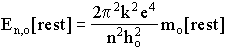3.1
where the subscript o means that the atom is at rest. When the hydrogen atom is given a velocity, the energy of each of the n levels changes as seen by an observer remaining at rest and using rest units.
We must notice that the frame in which the observer is actually located has no physical relevance. However, a description of the units (of mass, length and clock rate) used by the observer is necessary. Of course, one generally assumes that the observer uses the units that exist in his own frame. However, the description will be complete only when we specify the frame of origin of the units instead of assuming every time that the observer uses the units of his own frame.
The energy levels of the moving atom (using rest frame units) are given by putting equations 2.22 and 2.23 in equation 3.1. The Bohr equation becomes:3.2
Furthermore, since the Bohr radius ao of an atom at rest is:3.3
using equations 2.22, 2.23 and 3.3, the Bohr radius of a moving atom will be:3.4
This means that the Bohr radius ao increases linearly with g. This will be discussed in section 3.4. From equation 3.2, we see that the energy between atomic transitions of a moving atom (which determines the clock rate) decreases linearly as g increases (using the units of the rest frame). We conclude that according to quantum mechanics, the rate of a moving clock slows down when its velocity increases.
This is compatible with the slower clock rate of moving atoms as observed experimentally and interpreted erroneously as time dilation. The popular phrase "time dilation" should be interpreted as meaning that the rate of the moving clock has slowed down and not that time has dilated. Combining the Bohr equation (equation 3.2) with solely the mass relationship (equation 2.23) and neglecting equation 2.22 would lead to a rate increase of the moving clock. This is contrary to observations and to mass-energy conservation, as seen in chapter two. The correction due to mass-energy must be applied to the Planck parameter h as given by equation 2.22. Consequently, the observed slowing down of the clock rate of moving clocks, which is implied by equation 3.2, is an experimental confirmation of equation 2.22. This also solves the apparent contradiction presented in section 2.7.

3.3 - The Lorentz Equation for Time.
From the relativistic Bohr equation presented above, let us calculate the energy of an atom located on a stationary frame. From equation 3.1 we see that the energy states of a stationary atom (using rest frame units) are: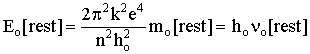3.5
where hono[rest] is the internal energy of excitation in the atom, using rest frame units. Due to its velocity, the atom located on the moving frame has a different internal energy. Equation 3.2 gives (using rest frame units):3.6
where honv[rest] is the internal energy of excitation of the moving atom (using rest frame units) that can possibly be received on a frame at rest in order to be compatible with mass-energy conservation. Consequently, the radiation emitted from such an atom has a lower absolute energy and frequency. This can be seen from equations 3.5 and 3.6: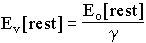3.7
From equation 3.7, we see that using rest units, there is less internal energy Ev[rest] in the moving atom (due to equation 2.22) than in the atom at rest (Eo[rest]).
The middle term of equation 3.6 represents the internal excitation energy of the moving atom in rest units while the right hand side term represents the same internal energy available that can be received by an observer at rest (also in rest units). Since the energy states of the moving atom have less energy (always in rest units), the observer at rest will detect a lower frequency (as measured using rest frame units) if that energy is emitted. We must notice that in both cases (equations 3.5 and 3.6), the constant h refers to a measurement done in the stationary frame (meaning that the measurement is made from a frame having zero velocity and using rest units) so that the parameter h must have the subscript o.
One must notice a fundamental physical mechanism implied in the decrease of internal energy in the hydrogen atom as given in equation 3.7 (using rest units). The internal potential energy in a hydrogen atom is given by equation 1.12. When the hydrogen atom is moving, equation 1.12 shows that due to the increase of velocity, the electron mass me and therefore the energy En increases by a factor g. However, at the same time, the Planck parameter which is squared and located at the denominator also increases. The overall effect is that the internal energy En in the atom decreases when the velocity increases. One must then realize that when the velocity increases, the electron mass becomes larger but the decrease of the Planck parameter corresponds to a decrease of the force between the electron and the proton.
From equations 3.5, 3.6 and 3.7 we obtain that the ratio between the clock rates of the moving clock and the clock at rest is:3.8
The last term nv[rest]/no[rest] of equation 3.8 gives the ratio between the frequencies (in rest units) of oscillation of two independent clocks having different velocities according to the Bohr equation. This relationship has nothing to do with the relative values of the frequencies of an electromagnetic wave as given in equation 2.21. In equation 3.8, there are two different frequencies emitted by two different clocks observed in a single frame. However, in the case of equation 2.21, we have a single clock emitting a single frequency observed by two independent observers located in different frames.
Let us consider figure 3.1 on which a moving clock M travels in front of a station (at rest) from A to B. Let us measure the difference of clock displays DCDo recorded on a clock located on the station at rest between the instants the moving clock M passes from A to B. We will also measure the difference of clock displays DCDv recorded on the moving clock while it passes from A to B. It is clear that the absolute time (as defined in section 2.3) is the same for M to pass from A to be B in both observations.Figure 3.1

However the two clocks will not display the same difference because they do not run at the same rate. The ratio between those two differences of clock displays DCDo and DCDv is proportional to the ratio of the clock rates no[rest] and nv[rest]. Therefore: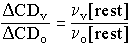3.9
Combining equation 3.9 with equation 3.8 gives:3.1
which is mathematically identical to:3.11
From the usual definition of g, equation 2.2: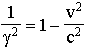3.12
we find that, using equation 3.11:3.13
Einstein made the hypothesis that "time is what clocks are measuring". This means that the Dt in Einstein's relativity and in the Lorentz equations is only a difference of clock displays on a clock at rest to which the units of time were given:
 DCDo = Dt 3.14
In reality, since Dt is nothing more than a DCD, the units of DCDo (which is a pure number) must be given to Dt. Let us give an example. It is believed that in Einstein's relativity and in the Lorentz equations, when an excited atomic state of a moving atom has not become de-excited after a classical time interval, it is because the time interval was shorter within the moving frame than in the rest frame. We have seen above that this explanation is incorrect and that the reason is that the principle of mass-energy conservation requires a change in the atom parameters and consequently, a slower internal motion inside atoms. This slower internal motion makes moving clocks function more slowly. Therefore, the Dt measured by Einstein's and Lorentz's clocks is not a time interval at all, but a difference of clock displays (DCD) of a clock running more slowly. The correct explanation is that when, in the Lorentz equation, we find that the Dt' is different from Dt during the same time interval, we are fooled by clocks running at different rates in different frames. It is an error of interpretation to give time units to Dt and Dt' in the Lorentz equations while they are no more than differences of clock displays as admitted by Einstein. Since the DCD is a pure number, the Dt in equation 3.14 is also a pure number. Similarly, the difference of cloc k displays DCDv is called Dt' in the Lorentz equations:
 DCDv = Dt' 3.15
A comparison with the Lorentz equations, as given with equations 3.14 and 3.15, is useful to examine some mathematical properties common to both interpretations. Equations 3.14 and 3.15 in equation 3.13 give:3.16
By definition, the number of units x representing the distance traveled during Dt (for Einstein corresponding to the time while a clock shows DCDo) is:
 x = vDt or x = vDCDo 3.17
Of course, x is not a real distance, as explained in section 3.1. Let us substitute Dt from equation 3.17 to the second term Dt of equation 3.16. We get:3.18
Equation 3.18 gives the relationship between Dt' (which is a difference of clock displays) displayed by a clock located at a distance x from the origin and moving at a velocity v and Dt displayed by a stationary clock. We observe that equation 3.18 is exactly the Lorentz equation for time and that it is compatible with Einstein's hypothesis that time is what clocks display. This equation is simply an exact mathematical description of mass-energy conservation in agreement with equations 2.22 and 2.23 and with the physical mechanism implied by equation 3.2 We notice finally that the Lorentz transformation for time has been demonstrated here without using the hypothesis of the constancy of the velocity of light nor any new hypothesis. We have used only the mass-energy relationship E = Km from equation 2.3. In fact, we have obtained the Lorentz equation for time without the use of any of Einstein's relativity principles.
One must conclude that the Lorentz transformation derived above is in reality a transformation of relative clock displays between frames. Then Dt and Dt' (when related to this Lorentz equation) represent differences of clock displays DCD.

3.4 - Length Dilation Due to Kinetic Energy.
Length dilation and contraction have been demonstrated in chapter one for matter placed in a gravitational potential. Using equation 3.4, we will now show that the Bohr equation also gives a change of length when matter acquires a velocity v. This will be done without involving the constancy of the velocity of light. According to equation 3.4, we have: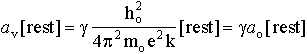3.19
Therefore, the relative size of the Bohr radius as a function of velocity is:3.2
Let us consider a reference meter made of ordinary classical atoms. We see from equation 3.20 that the size of atoms, which is proportional to the Bohr radius or to the interatomic distance (see Appendix I), increases as a function of velocity. This means that the size of all material matter increases with velocity.
We know that the number of atoms Na making up the length of a rod does not change with velocity. Furthermore, it is well established in modern physics that the interatomic distance jo is proportional to the Bohr radius ao so that jv[rest] = gjo[rest]. The length lo of a rod is:
 lo[rest] = (Na-1)jo[rest] 3.21
At velocity v, the length lv is:
 lv[rest] = (Na-1)jv[rest] = (Na-1)gjo[rest] 3.22
We note that the number of atoms Na is much larger than unity. Therefore, using equations 3.21 and 3.22 we have: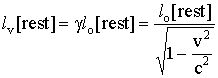3.23
Equation 3.23 shows that there is length dilation of matter when its velocity increases (in a constant gravitational potential). Length dilation is a real physical phenomenon involving no stress nor any pressure, similar to length dilation and length contraction in a gravitational field, as shown in chapter one. It is just the natural equilibrium of matter given by quantum mechanics that makes it dilate at relativistic velocities. Space dilation or space contraction is meaningless.
The fact that we are led from our reasoning to length dilation instead of length contraction does not represent a problem since the assumed phenomenon of length contraction has never been observed experimentally in special relativity. On the contrary, we need length dilation to be compatible with the slowing down of clocks, which is also required by quantum mechanics and has been observed experimentally. In order to be coherent with quantum mechanics and mass-energy conservation, one must understand that there exists no length (nor space) contraction in special relativity because g is always equal to or larger than one (equation 3.23). Only length dilation can be produced when there is an increase of velocity.

3.5 - The Lorentz Transformation for Lengths.
Let us consider two identical frames O-X at rest. The axis of those frames are constructed with many rods in series each having a length exactly equal to one reference meter (defined in section 2.4). A mass M is located at a distance x[rest] from the origin O[rest]. For a stationary observer using the reference meters located on the frame at rest, the coordinate of the mass M is:

 x[rest] = nometer[rest] 3.24
where no is the number of times the meter rod, when defined at rest (meter[rest]) must be used to form the length x[rest]. The symbol no is a pure number measured in the stationary (subscript o) frame. We must recall that contrary to Newtonian physics, the simple use of the number no is not sufficient to represent a length. A length must necessarily be represented by a pure number multiplied by the length of the reference meter.
Let us give the velocity V to one of the frames that we now call O'-X'. At time t = 0, the origin O' of the moving frame coincides with the origin O of the rest frame. The axis O'-X' is arbitrarily displaced on figure 3.2 in order to avoid confusion. Before the frame O'-X' acquired its velocity, the distance between the origin O and the mass M was identical in both systems. After the frame O'-X' has reached velocity V, we have seen that the Bohr radius and all physical material on the moving frame are dilated as given by equation 3.23. Therefore the reference meters used to form the axis are longer. The mass M' on the moving frame is fixed with respect to that frame and does not move with respect to the particular segment of meter where it is fixed. Therefore the number nv of those standard moving rods between M' and the origin O' is necessarily the same and no = nv.Figure 3.2

However, the absolute distance x'[mov] between M' and O' will increase because the length of the standard meter has increased due to the increase of the Bohr radius. The distance x'[mov] between M'[mov] and the origin O' is given by:

 x'[mov] = nvmeter[mov] = nometer[mov] 3.25
with
 nv = no 3.26
Using the notation x[rest] = lo[rest] and x'[rest] = lv[rest] equation 3.23 gives:
 x'[rest] = gx[rest] or Dx'[rest] = gDx[rest] 3.27
Equation 3.27 means that using rest frame units, the distance x' (which is O'-M') is g times longer than the distance x (which is O-M) also using rest frame units even if the numbers of local meters no and nv are the same. 3.5.1 - Apparent and Absolute Time.
In order to predict the consequences of the change of "clock" rate between systems, we must be able to compare predictions between different frames. Let us examine the relationship between the "apparent time" in different frames. In Einstein's relativity, the "time" is defined as what is perceived by each observer. It is equal to what a clock measures in its own frame. It is called t in the rest frame and t' in the moving frame.

Consequently, each frame has its own "time" but we know that it is only apparent. Real physical time does not flow faster because the local clock runs faster. For an observer at rest, Einstein's interpretation assumes that his "time" t is the one shown by his clock at rest. Similarly, the "time" t' is the apparent time in the moving frame. Since the moving clock runs at a different rate than the clock at rest (see equation 3.8), the time on the moving frame "appears" (as seen by an observer at rest) to elapse at a different rate giving:

 t ¹ t' 3.28
We define the "absolute second" So[rest] as the time interval t taken by the atomic clock at rest (located away from any gravitational potential) to record a constant number Ns of oscillations. Since that clock at rest runs at a frequency no[rest], the apparent rest second (called absolute second) will be elapsed when So equals unity. This gives:3.29
On a moving frame, the "apparent second" Sv[mov] is equal to the time taken by the local clock moving at velocity V to record the same number of oscillations Ns. Therefore during one "apparent second" (Sv) on the moving frame (at velocity V), by definition, the clock must record the same number of oscillations as the clock on the rest frame does during one "absolute second" (So). This means that during one "apparent second" inside any frame, the local DCD is always the same number. Then, since clocks have different rates, in different frames, the "absolute duration" of the "apparent second" varies with the velocity of the frame carrying the clock.
It is arbitrarily decided that the rest second (in zero gravitational potential) is called the "absolute second of reference". Since the number of oscillations is the same for any local second, we have, for the case of apparent second Sv in a frame moving at velocity:
 DCD(So)[rest] = DCD(Sv)[mov] 3.3
From the definition of apparent seconds in a frame moving at velocity V, with equations 3.29 and 3.30, we find that the duration of one moving second is:3.31
In order to be able to compare "apparent seconds" generated in different frames, we must be able to express the "apparent time" duration using common units. We have from equation 3.8:
 no[rest] = gnv[rest] 3.32
Equation 3.32 in equations 3.31 and 3.29 gives:3.33
Equation 3.33 shows that the unit of time Sv in the moving frame is g times longer than the unit of time So in the rest frame.
Let us consider the "real time intervals" corresponding to the same numerical value of local apparent "x" seconds elapsed in both the rest frame and the moving frame. The DCD shown by either clock is the same in both frames. In Einstein's relativity, this was erroneously interpreted as the same time interval in both frames. In the rest frame, the real time t[rest] is equal to the number of seconds "x" times the duration of the apparent second So at rest. This gives:
 t[rest] = xSo[rest] 3.34
In the moving frame, the real time (in rest units) is called t'[rest]. It is equal to the number "x" of seconds times the duration of the apparent moving second Sv:
 t'[rest] = xSv[rest] 3.35
Combining equations 3.33, 3.34 and 3.35 gives:
 t'[rest] = gt[rest] or Dt'[rest] = gDt[rest] 3.36
Equation 3.36 shows that when we consider the same number of local "apparent seconds" (i.e. the same difference of clock displays) in two different frames, the real absolute time spent on the moving frame is g times longer that the absolute time spent on the rest frame.
Equation 3.36 is equivalent to equation 3.18 when time is measured at the same location (x = 0). However, one must understand that the change of time between systems suggested by Einstein is only apparent because clocks in different frames run at different rates. This has erroneously been interpreted as time dilation in the past, but we see now that it is nothing else than clocks running at different rates in different frames.

3.5.2 - Relationship between Velocities V and V'.
On figure 3.2, the right hand side direction of the axes O-X and O'-X' is positive in both frames. When the moving frame O'-X' has a velocity toward the right hand side, the coordinate of the location M' increases (in time) with respect to the rest frame O-X. Therefore location M' has a positive velocity with respect to the rest frame O-X. However, figure 3.2 shows that when the moving frame (with origin O') travels to the right hand side, location M moves to the left hand side with respect to the frame O'-X'. The coordinate of location M is getting more and more negative (in time) with respect to the frame O'-X', while the coordinate of location M' is getting more positive in time with respect to the frame O-X. This means that the velocity V' of point M' (with respect to O-X) has the opposite sign of the velocity V of point M with respect to O'-X'. This result comes out of pure geometrical considerations illustrated on figure 3.2. Therefore:3.37
Equation 3.37 signifies that the velocities have opposite directions. We will show now that the velocities V and V' have the same magnitude.

3.5.3 - Relative Velocities within Systems.
Let us consider a rest frame and a moving frame. Both frames were identical before the moving frame started to move at velocity V[rest]. Inside both frames, we consider rods that had the same length when they were initially in the same frame at rest. This can be verified later if we count the same number of atoms in both frames for the length of either rods. The rod at rest extends from O to M and the moving rod extends from O' to M'.
There are at least two different ways to compare velocities between frames. One way consists of measuring directly the velocity in each frame using proper values and comparing numbers. Another way, the one we will use here, is to use a definition of velocity in each frame and to compare the corresponding elements of the definitions. The velocity u of a moving object across O-M with respect to the rest frame is defined as:3.38
With equation 3.38, we start dealing with a series of equations related to velocities. These velocities can have any direction in space and might be described by vectors. However, such a description would lead to a very heavy notation that could be confusing and would require useless efforts. This is avoided by defining that in every equation between 3.38 and 3.46, we consider that u and u' represent the magnitudes |u| and |u'| of these parameters. The appropriate mathematical sign of the velocities will be considered starting with equation 3.46.
Inside the moving frame, a similar slowly moving object moves from O' to M' (distance Dx'). During the time Dt' the slow moving object crosses the distance Dx' from O' to M'. The velocity of the slow moving object with respect to the moving frame is defined as:3.39
We have seen that, before the moving rod (O'-M') started to move, it was similar to the rod in the rest frame (O-M) and that both clock rates were similar. Consequently, we can use equations 3.27 and 3.36. Let us put the transformation of coordinates given by equations 3.27 and 3.36 into the equation 3.38. We get:3.4
Let us use equation 3.23 to calculate the ratio between the units of length. If the length lo is a unit of length equal to one meter using rest units, we see that this unit of length becomes glo on the moving frame. Therefore the relationship between the units of length is:
 lo[mov] = glo[rest] or meter[mov] = gmeter[rest] 3.41
This means that when we move from the rest frame to the moving frame, the unit of length becomes g times longer. Therefore, in order to represent the same physical length using longer units of length, the number of units Dx'[mov] must be smaller. This gives: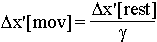3.42
In the case of time, a corresponding phenomenon takes place. Let us consider equation 3.36. We see that a time interval Dto equal to one unit of time in the rest frame becomes g times larger in the moving frame because it takes more time for the slower clock to show the same DCD. In that case, we see from equation 3.36 that the change of local units of time Dto between frames gives:
 Dto[mov] = gDto[rest] or sec[mov] = gsec[rest] 3.43
This means that when we move from the rest frame to the moving frame, the local unit of time becomes g times larger. Therefore in order to represent the same absolute time interval using longer units of time, the number of units Dt'[mov] must be smaller. This gives:3.44
Equations 3.39, 3.40, 3.42 and 3.44 give: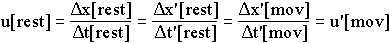3.45
Equation 3.45 shows that the velocity u measured using the rest frame units is the same as the velocity u' using the moving frame units.
Among the values of velocities which can be given to u, we can choose the velocity V which is the velocity of the moving frame with respect to the rest frame (rest frame units). Symmetrically, let us call V' the velocity u' of the rest frame with respect to the moving frame (using moving frame units). Using equations 3.37 and 3.45 gives:
 V = -V' 3.46
or
 Vo[rest] = -Vv[mov] 3.47
Equation 3.46 shows that the proper value of the velocity of the moving frame with respect to the rest frame is the same (negative) as the proper value of the velocity of the rest frame with respect to the moving frame.
Let us add that a velocity appears as a physical concept for a physicist. However, we have seen above that a comparison of velocities in two different frames having a relative velocity leads to the same numbers. We have seen that when we are in a moving frame, the ratio between the distance traveled and the time taken to travel it changes with respect to the rest frame. Both the numerator (the distance) and the denominator (time interval) change by the same ratio. Consequently, a constant velocity is nothing more that a constant ratio between two fundamental physical quantities. On can say that the constant velocity in different frames means the same thing as three oranges out of six is the same thing as four apples out of eight. Velocities are just ratios of physical quantities.

3.5.4 - Lorentz's Second Relationship.
In order to find the dynamical relationship between the coordinates x' and x, let us now combine the quantities x, V and t calculated above. In classical mechanics inside the moving frame we have:

 x' = xo'+V't' 3.48
where xo' is the coordinate x at t = 0 and V' is the velocity between frames. In order to be more specific, in complete notation, equation 3.48 should be:
 xv[mov] = xov[mov]+Vv[mov]tv[mov] 3.49
Let us consider first in equation 3.49 the expression tv[mov]. The term tv represents the number of units that is multiplied by the length of the unit [mov]. Let us calculate what would be the quantity tv[mov] using the [rest] units of length instead of the [mov] units of length.
From equation 3.44, we have:
 tv[rest] = gtv[mov] 3.5
In the case of the units of distance (xv or xov) we use again the same method. With the help of equation 3.42 we find:
 xv[rest] = gxv[mov] 3.51
and
 xov[rest] = gxov[mov] 3.52
From equation 3.49, transforming xv[mov] with 3.51, xov[mov] with 3.52, and tv[mov] with 3.50, we get after multiplying both sides by g:
 xv[rest] = xov[rest]+Vv[mov]tv[rest] 3.53
From equation 3.53, transforming xov[rest] with 3.27, Vv[mov] with 3.47, and tv[rest] with 3.36, we get:
 xv[rest] = g(xoo[rest]-Vo[rest]to[rest]) 3.54
Using a more conventional notation this is:
 x' = g(x-Vt) 3.55
Equation 3.55 gives the relationship between the coordinate x' on the moving frame and the coordinate x, the velocity V and the time t on the rest frame. This relationship results solely from mass-energy conservation and quantum mechanics without using any of Einstein's relativity principles. However, equation 3.55 is exactly identical to the Lorentz equation related to lengths. The demonstrations leading to equations 3.18 and 3.55 show the uselessness of Einstein's special relativity principles. Most importantly, this demonstration provides a way to give a logical interpretation to experiments without space or time contraction or dilation.

3.6 - Constant Velocity of Light within Any Frame of Reference.
We must notice that c is also a velocity obtained from the quotient of a distance by time within any frame. Let us consider that the internal velocity u is the velocity of light c. In the moving frame, the velocity u' equals c'. Therefore when the velocities u and u' considered are applied to light, equation 3.45 gives:

 c = c' 3.56
When we use the complete notation, we get:
 cv[mov] = co[rest] 3.57
This means that following equations 3.45 and 3.56, one must conclude that the physical mechanism resulting from mass-energy conservation and quantum mechanics leads to the conclusion (not the hypothesis) that any velocity, including the velocity of light, is constant as measured within any frame (using proper values). Contrary to Einstein and Lorentz, we do not have to make the arbitrary hypothesis that the velocity of light is constant inside all frames. We have found that the constancy of the velocity of light is a necessary conclusion to mass-energy conservation and the quantum mechanical equations.
From another point of view, the value of c, called the velocity of light, has been defined in section 2.4 as the square root of K (the quotient between energy and mass) which is the fundamental basis of mass-energy equivalence. Any theory or experiment not compatible with the constancy of the velocity of light (using proper values) is therefore necessarily not compatible with quantum mechanics and mass-energy conservation. However, since the velocity of light is given as the quotient of two quantities (length and DCD) that are different in different frames, the physical meaning of that constant ratio is subtle.

3.7 - Non-Reality of Space Dilation, Contraction or Distortion.
The distance Dx traveled in a time interval Dt is defined as:

 Dx = vDt 3.58
Let us assume an observer traveling between the ends of a long stationary rod having a length Dx. That length Dx is calculated from the velocity v times the time interval Dt necessary to travel between the ends of the rod. We know that the velocity v is the same on any frame. However, the difference of clock displays DCDo (which is interpreted as time Dt by Einstein) on the rest frame is different from DCDv (interpreted by Einstein as time interval Dt') on the moving frame. Consequently, according to Einstein's interpretation, the length Dx' measured by the moving observer is different from the length Dx of the same rod measured by the observer at rest. At the velocity of light, the DCDc decreases to zero so that the (apparent Einstein's) length Dx' becomes zero for the moving observer because his moving clock has stopped running.
It is irrational to claim that the length of the stationary rod changes and even becomes zero just because the observer changes his velocity. How can the length of a rod logically change because a non interacting observer looks at it? The rod would become longer or shorter depending on the observer's own velocity. The length (and other properties) of the rod would not be a property that would belong to matter. It is the observer that would set the length of the rod and different observers would simultaneously find different lengths for the same rod depending on their observing conditions. Then, what would be the length of the rod if there were no observer? It is just like the statement that the moon is not there when nobody is looking at it. We believe that this is nonsense and that the length of matter is independent of the observer. This is the same irrationality that appears in quantum mechanics and which has already been discussed .
We have not yet defined how to measure space. This is because space is not measurable unless we fill it up at least partially with matter. Then, it is that matter that we measure, not space. Whether space is empty or full of matter, we generally refer to it as "space". We know several methods of measuring lengths of objects but there does not exist any method of measuring space without using matter as a reference. In relativity, space is often referred to as being contracted or dilated. How can it be contracted or dilated when there is no method of measuring it without assuming some matter in it? The properties of matter are then inadvertently attributed to or confused with space. The same comment applies to the belief of space distortion. How can there be space distortion when we cannot measure space directly in the absence of matter? The interpretation of space distortion is nothing more than a change of the Bohr radius in the measuring instrument or in the matter filling the space.
This problem is easily solved logically when we consider that the internal atomic mechanism of the observer runs at a different rate since electrons in motion have a different mass. This has nothing to do with the illusion of space dilation or distortion. One must conclude that the expressions "space contraction" and "space distortion" are irrational. They bring confusion and must be eliminated.

3.8 - Transformation of Units in Different Frames.
There are many other consequences to the relativistic changes of lengths and masses. For example, in chapter one we have seen that the mass of particles decreases when located at rest in a lower gravitational potential. In chapter three we have seen that masses increase with velocity due to the absorption of kinetic energy. This means that if we take an object of one kilogram on Earth and move it to a location at rest on the solar surface, about one millionth of its mass will disappear and be carried away by the energy generated during the slowing down of the object falling into the Sun. Even if there is exactly the same number of atoms in one Earth kilogram after it is carried on the Sun's surface, we see that the solar kilogram has less mass than the Earth kilogram using any common frame of comparison of mass units. Consequently, there is more energy (in Earth joules) in one Earth kilogram than in one solar kilogram. This is required by the principle of mass-energy conservation.
Similar considerations must be applied to most physical constants. Because of the principle of mass-energy conservation, the units must always be specified (kg[Earth], meter[Earth], joule[Earth], second[Earth]). However, the electric charge appears to be constant in any frame. This means that the ratio of the electron charge divided by the electron mass (e/m) is different in different frames. For example, e/m is smaller on Earth (when using Earth units) than on the solar surface (using Earth units). In order to be able to compare those quantities with the ones calculated in different frames, we must take into account the difference of gravitational potential or the difference of kinetic energy. To define accurately the reference kilogram, the reference meter, etc., we must know the exact altitude on Earth at which these units have been defined.

3.9 - Failure of the Reciprocity Principle.
We have studied above some of the differences existing between a frame in motion and a frame at rest. In a moving frame, clocks run at a slower rate, the Bohr radius is larger and so are masses because of their kinetic energy. Let us consider a body on the rest frame having a mass mo[rest]. Its total energy is:

 Eo[rest] = mo[rest]c2 3.59
When mo[rest] is accelerated to velocity vo[rest] with respect to the rest frame, its mass becomes mv[rest]. We get: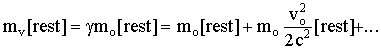3.6
Equation 3.60 shows that the moving mass mv[rest] is larger than the rest mass mo[rest]:
 mv[rest] > mo[rest] 3.61
Let us consider now a train moving at velocity vo[rest] carrying an observer and the mass mentioned above. The mass of the train, of the observer and of the body described above becomes g times larger than when at rest. However, since the units in the moving train have been modified by the same ratio g, the changes of mass, clock rate and length are undetectable to the moving observer, even if they are real. Inside the moving train, an observer using Einstein's reciprocity principle will claim that the object of mass mv[rest] is at rest with respect to him. He will thus call it Mo[rest]. Therefore:
 Mo[rest] º mv[rest] = gmo[rest] 3.62
It is because we use Einstein's hypothesis of reciprocity that we write [rest] after Mo in equation 3.62, since Einstein's hypothesis assumes that the mass that has been transferred to the train is now at rest for the observer moving with the train. Furthermore, the symbol º used in equation 3.62 does not mean that we are defining a new quantity. The symbol º means that Mo is the same object in the same physical condition as mv[rest].
Now, the moving observer takes the object of mass Mo[rest] (that is stationary with respect to him) and throws it at velocity vo[rest] with respect to his moving train (considered at rest in his frame) in the direction opposite to the direction of motion of the train. According to Einstein's principle of reciprocity, the mass projected at velocity vo[rest] with respect to the moving frame acquires velocity and energy with respect to the moving frame (now considered at rest). Einstein's principle of reciprocity says that all frames are identical which means that mass Mo[rest] increases when accelerated with respect to the train to become Mv[rest]. In fact, the reciprocity principle implies that the passage of the object of mass Mo[rest] from zero velocityo[rest] to vo[rest] (with respect to the train) increases its mass by g times, independently of the direction of the velocity of the mass with respect to the train. This gives:
 Mv[rest] = gMo[rest] 3.63
As expected from the relativity principle, equation 3.63 shows that mass Mv[rest] is larger than Mo[rest] giving:
 Mv[rest] > Mo[rest] 3.64
A physical representation of these changes of velocity shows that the mass Mv[rest] now has zero velocity with respect to the rest frame. It is back at rest on the rest frame. Mass Mv[rest] is then physically undistinguishable from mass mo[rest] since it is the very same object having the same zero velocity with respect to the same rest frame. Therefore physically, we must have:
 Mv[rest] º mo[rest] 3.65
Combining equations 3.62, 3.63 and 3.65 gives:
 mo[rest] º Mv[rest] = g2mo[rest] 3.66
Obviously, equation 3.66 is correct only if g equals unity so that the velocity must always be zero. This shows that the principle of reciprocity cannot be valid when we apply the principle of mass-energy conservation. We must conclude that Einstein's reciprocity principle is not coherent.
Contrary to Einstein's claim, the energy given to a mass accelerated with respect to the train must depend on the direction of its velocity with respect to the direction of the velocity of the train. When the directions are opposite, the two velocities (whose magnitudes are equal) cancel out and the mass of the body must come back to its original value in the rest frame. Otherwise we would discover that atoms of matter having traveled to another frame would have a different mass after their return to the initial frame. We must conclude that two frames cannot be equivalent when there exists a relative motion between them.

3.10 - References.

 P. Marmet, Absurdities in Modern Physics: A Solution, ISBN 0-921272-15-4, Les Éditions du Nordir, c/o R. Yergeau, 165 Waller, Ottawa, Ontario K1N 6N5, 144p. 1993.

3.11 - Symbols and Variables.

 ao[rest] Bohr radius at rest in rest units av[rest] Bohr radius in motion in rest units DCDo difference of clock displays on a clock at rest DCD(So)[frame] DCD corresponding to an apparent second in any frame DCDv difference of clock displays on a clock in motion En,o[rest] = Eo[rest] energy of the Bohr atom at rest in state n in rest units En,v[rest] = Ev[rest] energy of the Bohr atom in motion in state n in rest units ho[rest] Planck parameter on the rest frame in rest units hv[rest] Planck parameter on the frame in motion in rest units lo[rest] length of a rod at rest in rest units lv[rest] length of a rod in motion in rest units no[rest] clock rate of a clock at rest in rest units Ns number of clock oscillations in an apparent second nv[rest] clock rate of a clock in motion in rest units (So)[rest] definition of the absolute second in rest units (Sv)[rest] duration of one moving second in rest units u[rest] definition of the velocity in the rest frame in rest units u'[rest] definition of the velocity in the moving frame in rest units V = Vo[rest] velocity of M with respect to the moving frame in rest units V'= Vv[mov] velocity of M' with respect to the rest frame in motion units x[rest] distance between O and M in rest units x'[mov] distance between O' and M' in motion units
<><><><><><><><><><><><>

Chapter2  Contents    Chapter 4